﻿ 介孔二氧化硅固化柴翘挥发油及其粉体学性质的研究
 药学学报2019, Vol. 54Issue (8): 1493-1501     DOI: 10.16438/j.0513-4870.2019-0235PDF

1. 上海中医药大学中药学院, 上海 201203;
2. 上海中医药大学中药现代制剂技术教育部工程研究中心, 上海 201203

Mesoporous silica solidifying volatile oil from Bupleuri radix and forsythiae fructus and its micromeritic properties
TIAN Juan-juan1, YU Ya-ting2, ZHAO Li-jie2, FENG Yi2, WANG You-jie2, SHEN Lan11. School of Pharmacy, Shanghai University of Traditional Chinese Medicine, Shanghai 201203, China;
2. Engineering Research Center of Modern Preparation Technology of Traditional Chinese Medicine, Ministry of Education, Shanghai University of Traditional Chinese Medicine, Shanghai 201203, China
Key words: mesoporous silica     volatile oil     micromeritic property     physical characterization     stability

β-蒎烯吸附率的计算  取VO-DLP适量, 精密称定, 置具塞锥形瓶中, 精密加入无水乙醇25 mL, 称定重量, 超声处理10 min后, 放冷, 再次称重, 用无水乙醇补足减失的重量, 摇匀, 滤过, 取续滤液, 按气相色谱条件测定其中β-蒎烯的量并记为实际检测量; 另取挥发油110 mg于25 mL棕色量瓶中, 用无水乙醇定容, 摇匀, 通过色谱分析及相关计算求得固化粉末中β-蒎烯理论含有量, 并按公式(1)计算β-蒎烯吸附率[13-15]

 $\beta - {\rm{蒎烯吸附率 = }}\frac{{{\rm{固化粉末中}}\beta - {\rm{蒎烯实际检测量}}}}{{{\rm{固化粉末中}}\beta - {\rm{蒎烯理论含有量}}}} \times 100\%$ (1)

 ${\rm{tan}}\theta = \frac{h}{r}$ (2)
 ${\rm{CI}} = \frac{{{\rho _{bt}} - {\rho _b}}}{{{\rho _{bt}}}} \times 100{\rm{\% }}$ (3)
 ${\rm{HR}} = \frac{{{\rho _{bt}}}}{{{\rho _b}}}$ (4)
 ${\varepsilon _t} = 1 - \frac{{{\rho _b}}}{{{\rho _t}}}$ (5)

 ${E_{{\rm{SP}}}} = \frac{{{E_2}}}{m}$ (6)
 ${\rm{TS}} = \frac{{2F}}{{{\rm{ \mathsf{ π} }}DT}}$ (7)
 ${\rm{FES}} = \frac{{{H_1} - {H_2}}}{{{H_2}}} \times 100{\rm{\% }}$ (8)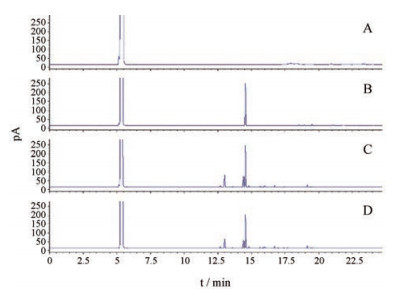Figure 1 Gas chromatogram of blank solvent (A), standard solution of beta-pinene (B), volatile oil (C) and volatile oil drug-loading powder (D)
2 VO-DLP的β-蒎烯吸附率及热稳定性Table 1 Adsorption rate and thermal stability of volatile oil drug-loading powder (VO-DLP) (n = 3, x ± s). Ex: Excipient (Sylysia 350FCP)
3 样品表征 3.1 外观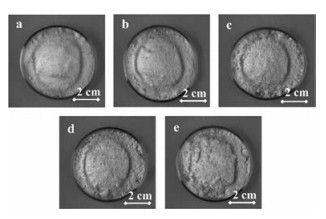Figure 2 Appearance of mesoporous silica Sylysia 350FCP (Sylysia 350FCP) (a), VO:Ex 1:4-DLP (b), VO:Ex 1:2-DLP (c), VO:Ex 1:1-DLP (d), VO:Ex 3:2-DLP (e)
3.2 扫描电镜分析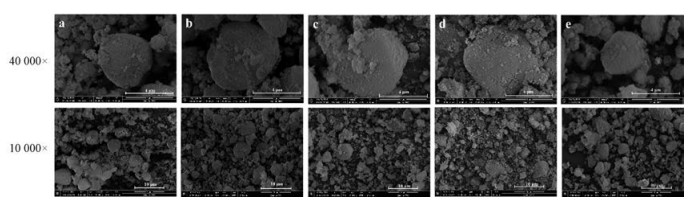Figure 3 The scanning electron micrograph of Sylysia 350FCP (a), VO:Ex 1:4-DLP (b), VO:Ex 1:2-DLP (c), VO:Ex 1:1-DLP (d), VO:Ex 3:2-DLP (e) (40 000 ×, 10 000 ×)
3.3 粉末X-射线衍射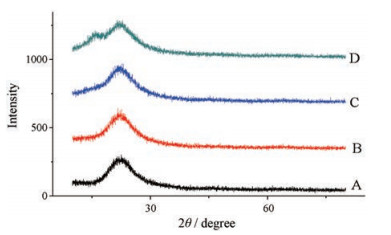Figure 4 The powder X-ray diffraction pattern of Sylysia 350FCP (A), VO:Ex 1:4-DLP (B), VO:Ex 1:2-DLP (C), VO:Ex 1:1-DLP (D)
3.4 同步热分析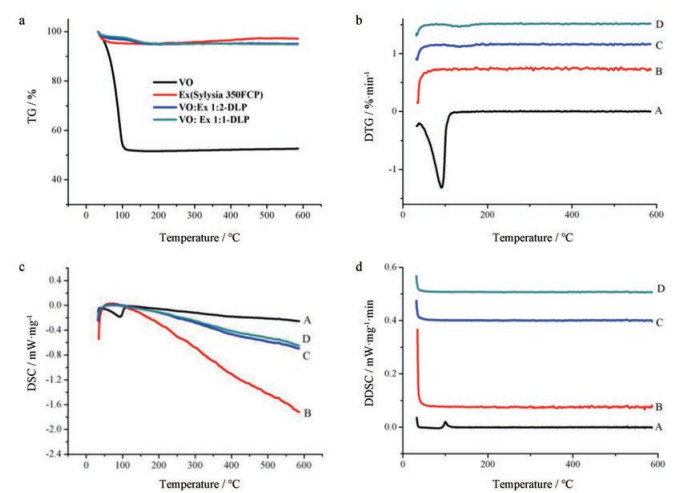Figure 5 Thermal gravity (TG), differential thermogravimetry (DTG), differential scanning calorimetry (DSC) and differential of DSC (DDSC) curves of volatile oil (A), mesoporous silica (B) and volatile oil drug-loading powder (VO:Ex 1:2-DLP as C and VO:Ex 1:1-DLP as D). a: TG curve; b: DTG curve; c: DSC curve; d: DDSC curve
4 粉体学性质考察Table 2 The physical properties of powders (n = 3, x ± s). ρb: Bulk density; AR: Angle of repose; ρbt: Tap density; ρt: True density; CI: Carr's index; HR: Hausner ratio
5 体外溶出度考察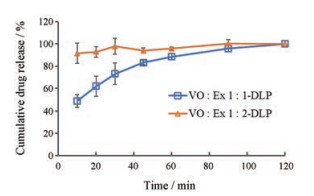Figure 6 The cumulative release percentage of VO-DLP with different drug loads (n = 6, x ± s)
6 压缩成型性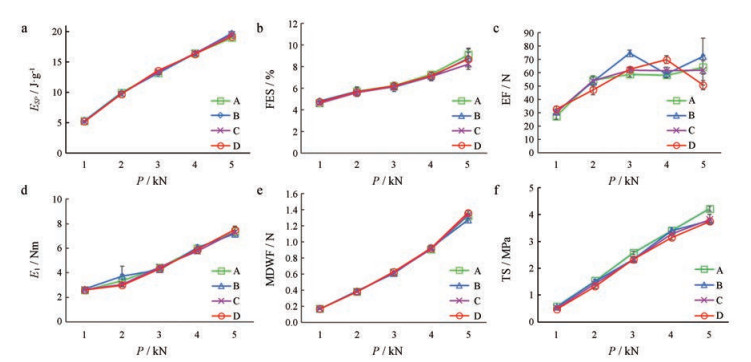Figure 7 The compressibility and compactibility of blended powder under different pressure: MCC-102+1% Sylysia 350FCP (A), MCC-102+1% VO:Ex 1:4-DLP (B), MCC-102+1% VO:Ex 1:2-DLP (C), MCC-102+1% VO:Ex 1:1-DLP (D). a: Esp - pressure curve; b: FES - pressure curve; c: EF - pressure curve; d: E1-pressure curve; e: MDWF-pressure curve; f: TS-pressure curve (n = 5, x ± s). ESP: Net energy per unit of quality; FES: Fast elastic stretch; EF: Ejection force; E1: Friction energy; MDWF: Middle die wall force; TS: Tensile strength
7 机械稳定性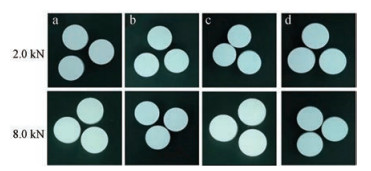Figure 8 Appearance characteristics of tablets of blended powder under 8.0 kN high pressure: MCC-102+1% Sylysia 350FCP (a), MCC-102+1% VO：Ex 1：4-DLP (b), MCC-102+1% VO：Ex 1：2-DLP (c), MCC-102+1% VO：Ex 1：1-DLP (d)
8 固化粉末的应用——工业化生产Table 3 Quality inspection of tablets prepared under 2.0 kN pressure